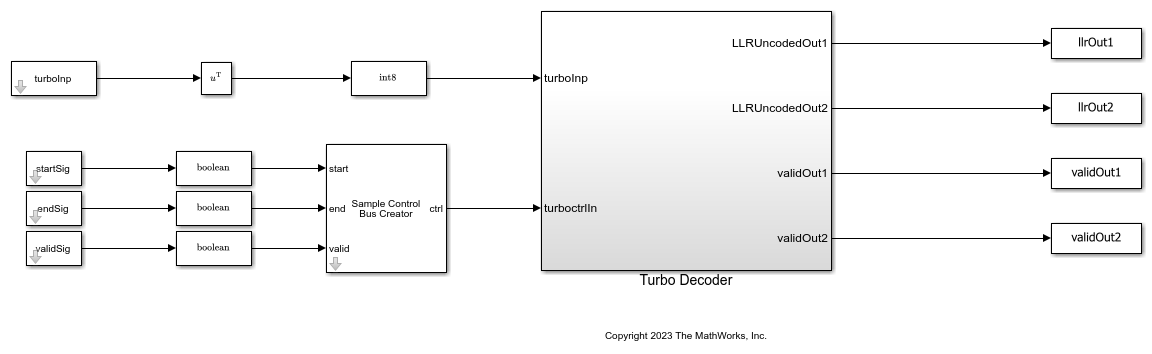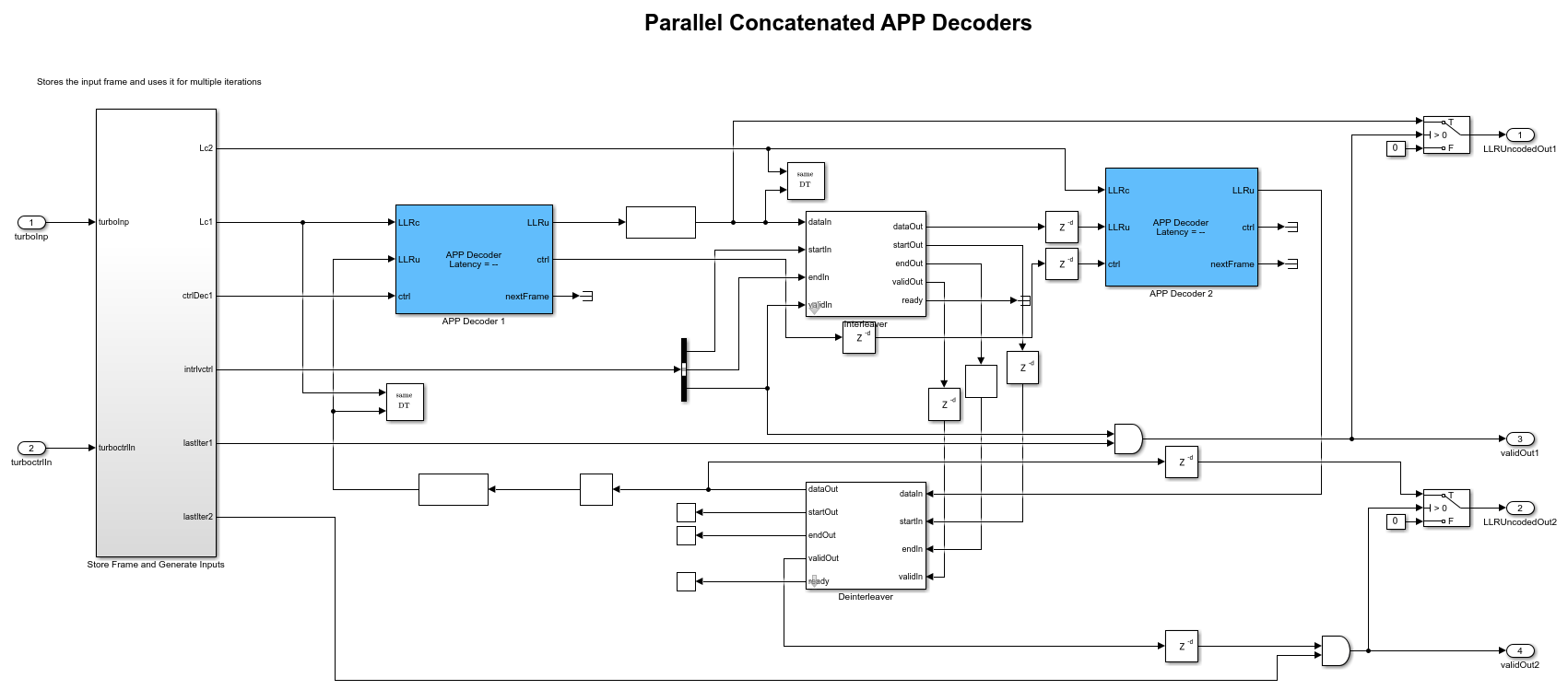# Parallel Concatenation Using APP Decoder

This example shows how to decode parallel concatenated convolution codes using the APP Decoder block. This example contains a Simulink® model that contains the basic structure of a turbo decoder with a rate of 1/3, constructed using two APP Decoder blocks.

### Introduction

The invention of turbo codes [ 1 ], along with the development of iterative decoding principles with near Shannon limit performance, has led to their integration in a wide variety of applications. Some of the applications include deep space communications, third generation wireless standards, and digital video broadcasting [ 2 ]. You can achieve iterative decoding by using two APP Decoder blocks and by having the encoders send the output soft information to each other forming a feedback loop. The block diagram shows the conceptual design of a turbo decoder for one iteration.### Model Architecture

This figure shows the top level model architecture of a turbo decoder. The model accepts three samples at a time with the corresponding start, end, and valid control signals.

```modelName = 'HDLParallelConcatenation'; open_system(modelName); ```Turbo Decoder

The following figure shows how the APP Decoder blocks are connected in parallel to build a turbo decoder. The output from the first decoder is interleaved and provided as an input to the second decoder and the output from the second decoder is de-interleaved and provided as an input to the first decoder forming a feedback loop. The Interleaver and Deinterleaver blocks are implemented based on the HDL Interleaver and Deinterleaver example. The `Store Frame and Generate Inputs` subsystem stores the frame and provides input to both the decoders based on the number of iterations. The `stopTime` value is adjusted so that the model runs for the specified number of frames, number of iterations, and frame length.

```load_system(modelName); open_system([modelName '/Turbo Decoder']); ```### Set Input Variables

Create a trellis and specify the input parameters as shown in the following code. You can change the variable values in this section based on your requirements.

```codeGen = [13 15]; % Specify code generator as a row vector of octal values. feedbackConn = 13; % Specify feedback connection as a scalar and an octal value. algorithm = 'Max'; % Specify the algorithm as 'Max' or 'Max*'. winLen = 64; numFrames = 10; frameLength = 1000; numIterations = 6; rowforInterleave = 4; snrRange = 0:6; orderOfQAM = 16; % Specify the QAM modulation order as 4, 16, 64, or 256 % Configured variables with the specified input parameters. These variables % are read-only. codeRate = length(codeGen); DecCodeGen = oct2dec(codeGen); K = length(dec2bin(DecCodeGen)); trellisStructure = poly2trellis(K,codeGen,feedbackConn); numOutputs = log2(trellisStructure.numOutputSymbols); numTailBits = 2*numOutputs*(K-1); if (numTailBits/3)-floor((numTailBits/3))==0 numTailMul3 = numTailBits/3; else numTailMul3 = ceil(numTailBits/3); end datasize = frameLength + numTailMul3; numberOfBits = numFrames*frameLength; latency = 2*(winLen) + 3 + 2+(2^(K-1) - 1); reShapedIndices = reshape(1:frameLength,[],rowforInterleave)'; interlvrIndices = reshape(reShapedIndices,1,frameLength); turboenc = comm.TurboEncoder(trellisStructure,interlvrIndices); stpTimeForOneIter = (2 + latency + datasize) + (datasize + 12) + (datasize + latency +12); frameGap = (2*(datasize+latency+12+1)+1)*(numIterations); inp2enc = zeros(frameLength,numFrames); turboDec = comm.TurboDecoder('TrellisStructure', trellisStructure, 'InterleaverIndices', interlvrIndices, ... 'NumIterations', numIterations,'Algorithm',algorithm); if strcmp(algorithm,'Max') algo = 'Max Log MAP (max)'; else algo = 'Log MAP (max*)'; %#ok<UNRCH> end stopTime = stpTimeForOneIter*(numIterations+1)*(numFrames); load_system(modelName); set_param([modelName '/Turbo Decoder/APP Decoder 1'],'TermMode','Terminated'); set_param([modelName '/Turbo Decoder/APP Decoder 2'],'TermMode','Terminated'); set_param([modelName '/Turbo Decoder/APP Decoder 1'],'Algorithm',algo); set_param([modelName '/Turbo Decoder/APP Decoder 2'],'Algorithm',algo); ```

### Generate Input Data and Simulate Reference Function Using MATLAB

Generate the input data and simulate the reference function in MATLAB using the following steps:

• Generate random binary data.

• Encode the data using the `turboenc` function.

• Modulate the data using the `qammod` function.

• Pass the modulated signal through an AWGN channel.

• Demodulate the received signal using `qamdemod` to give out approximated LLR values.

• Use the `comm.TurboDecoder` object to decode the LLR values and compute the bit error rate.

```rng("default"); for snrIdx=1:length(snrRange) ```
```simulink_input=[]; for fr=1:numFrames % Create random binary inputs inp2enc(:,fr) = randn(frameLength,1)>1; % Encode data using turbo encoder encdata = turboenc(inp2enc(:,fr)); % Modulate data modulatedData = qammod(double(encdata), orderOfQAM, 'InputType', 'bit', 'UnitAveragePower', true); % Add noise snrdB = snrRange(snrIdx); noiseVar = 10^-(snrdB/10); rxSig = awgn(modulatedData,snrdB,'measured'); % Demodulate data demod(:,fr) = -qamdemod(rxSig, orderOfQAM, 'OutputType', 'approxllr', 'UnitAveragePower', true,'NoiseVariance',noiseVar); %#ok<*SAGROW> % Decode data using turbo decoder function decodedBits(:,fr) = turboDec(demod(:,fr)); end matlab_output=decodedBits(:); matlab_output=matlab_output';% convert to a row vector % Reshape input to row vector matlab_input = reshape(inp2enc,[],numberOfBits); % Calculate bit error rate (BER) BER_matlab(1,snrIdx) = sum(xor(matlab_output,matlab_input))/numberOfBits; ```

### Run Model

Reshape the `qamdemod` function output and provide it as input to the `HDLParallelConcatenation` model. Generate the `start`, `valid`, and `end` signals based on the number of iterations and the latency of the APP Decoder block. Run the model and capture the bit error rate from the output signals `LLRUncodedOut1` and `LLRUncodedOut2`.

```inp2Sim = []; startSig = []; endSig = []; validSig = []; demodTerm = zeros(3*datasize,numFrames); demodTerm(1:size(demod,1),1:size(demod,2)) = demod; for fr =1:numFrames % Generate input signals to Simulink inp2Sim = [inp2Sim;demodTerm(1:3*datasize,fr);zeros(3*frameGap,1)]; %#ok<*AGROW> startSig = [startSig;true; false(datasize-1,1);false(frameGap,1)]; % Start signal endSig = [endSig;false(datasize-1,1); true;false(frameGap,1)]; % End signal validSig = [validSig;true(datasize,1);false(frameGap,1)]; % Valid signal end turboInp = reshape(inp2Sim,3,[]).'; % Run Simulink model sim(modelName); % Collect outputs from Simulink totaluncodedoutput1 = llrOut1(validOut1); totaluncodedoutput2 = llrOut2(validOut2); LLRu_out_sim = totaluncodedoutput1+totaluncodedoutput2; simulink_output = LLRu_out_sim>=0; % Calculate bit error rate BER_simulink(1,snrIdx) = sum(xor(simulink_output.',matlab_input))/numberOfBits; ```
```end ```

### Verify and Plot Results

Collect the bit error rate from MATLAB reference and Simulink model outputs and plot the results to show the signal-to-noise ratio (SNR) versus bit error rate (BER) performance.

```semilogy(snrRange,BER_matlab,'--o'); hold on semilogy(snrRange,BER_simulink,'-s'); grid on legend('MATLAB output','Simulink output'); xlabel("Signal-to-Noise Ratio (in dB)"); ylabel("Bit Error Rate (BER)"); title("Turbo decoding of 16 QAM with floating- and fixed-point data"); ```### Generate HDL Code

To check and generate HDL for this example, you must have an HDL Coder™ license. Use the `makehdl` and `makehdltb` commands to generate the HDL code and test bench for the `Turbo Decoder` subsystem.

Synthesize the `Turbo Decoder` subsystem on a Xilinx® Zynq®-7000 ZC706 evaluation board. The frequency obtained after place and route is about 235 MHz. The following table shows the post place and route resource utilization results for an 8-bit LLR input.

```F = table(... categorical({'Slice LUT';'Slice Registers';'BRAM';'DSP';}) ,... categorical({'6544';'5007';'8';'0'}), ... 'VariableNames',{'Resources','Usage'}); disp(F); ```
``` Resources Usage _______________ _____ Slice LUT 6544 Slice Registers 5007 BRAM 8 DSP 0 ```

### References

1. Berrou, C., and A. Glavieux. "Near Optimum Error Correcting Coding and Decoding: Turbo-Codes." IEEE Transactions on Communications 44, no. 10 (October 1996) : 1261-71. https://doi.org/10.1109/26.539767.

2. Schlegel, Christian, and Lance Perez. Trellis and Turbo Coding. IEEE Press Series on Digital & Mobile Communication. Hoboken, NJ: IEEE Press, 2004.